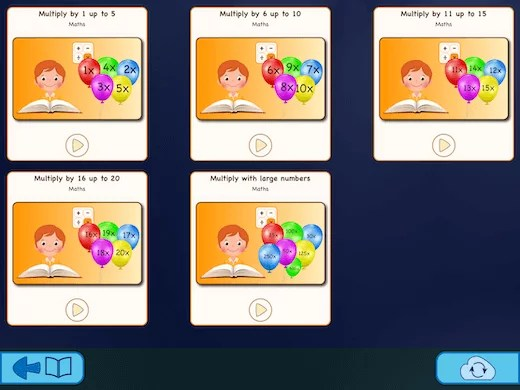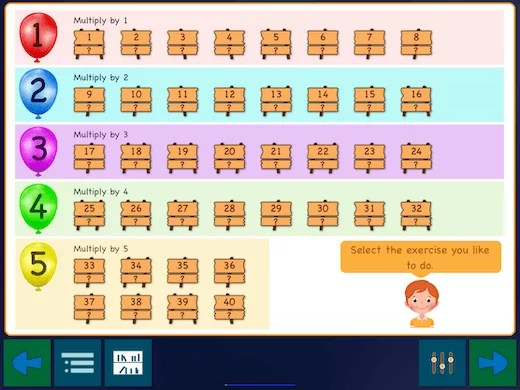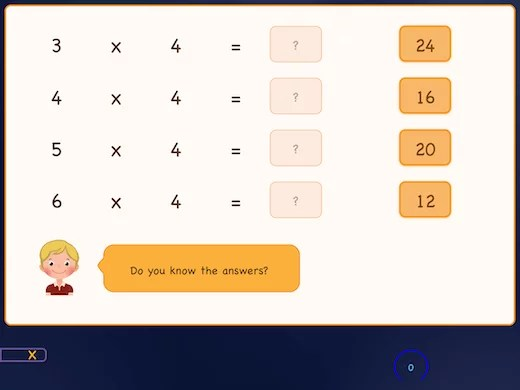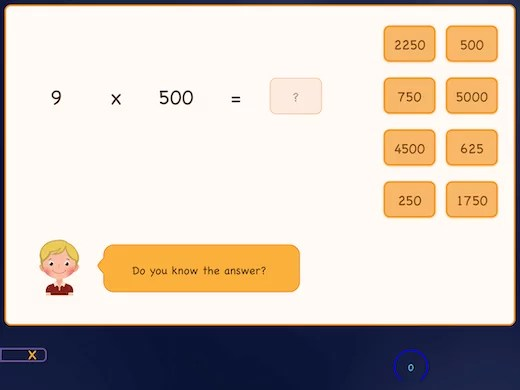Select Page# Learn multiplication times tables1 to 500

## Learn all the multiplication times tables 1 to 500 by practising

With this app, you learn the essential multiplication tables from your head in a simple way. This app contains many exercises in which you learn to multiply by the numbers 1 through 20 in an interactive way. Besides, the app also includes activities to learn to increase by 25, 30, 50, 100, 125, 250, and 500.
For each table, you can find eight exercises in the app.

The practice begins with learning the table in ascending order; then, you practice the tables in random order.
Finally, you practice the table again together with other times tables, which you have already learned previously.### Choose one out of the five booklets

This app contains five digital practice books. Each booklet contains about 40 exercises and will cover five times tables. Now you can learn in 5 steps the multiplication times tables 1 to 500 easily.First, you learn each time table in order. Then, you will discover them in random order.### Make the right choice!

It will be comfortable in the beginning because you get a limited number of answer options. However, it becomes more and more difficult as the answer options increase and you have to make a choice from right and wrong answers.### Learn multiplication sums separately

In the end, the exercises focus more on multiplying two numbers and not so much on the format of the table. It also combines many different times tables. This way, you also learn about the relationship between the tables.